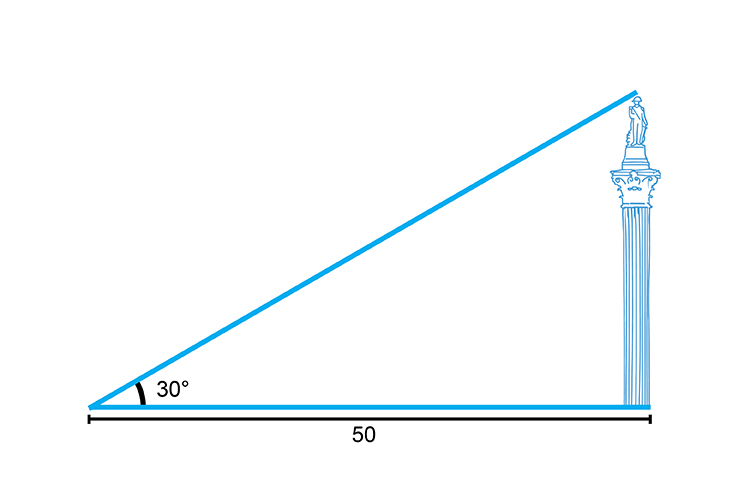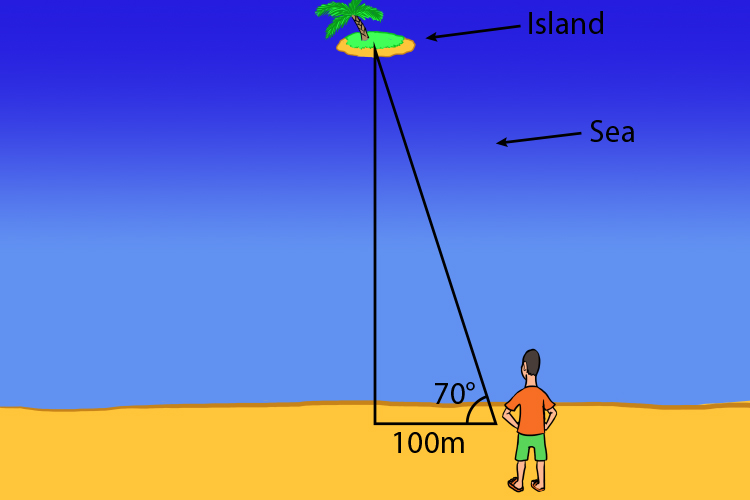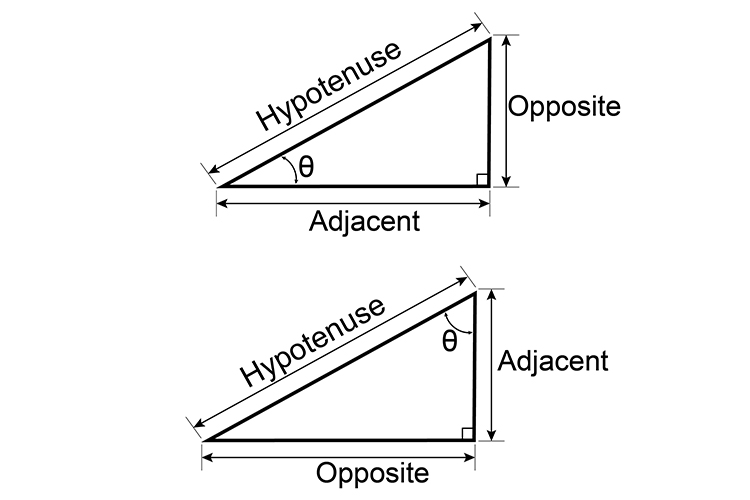# Trigonometry – Sin Cos Tan

## Trigonometry – is the relationship between the sides and angles of triangles.Sohcahtoa the great Native American war chief was very trigger-happy (trigonometry).

And then you can write down:

SOH    CAH    TOA

Pronounce          So – as in so what

Ca – as in cat

Toa – rhymes with boa (the snake)

In any right-angled triangle (90°), if we know:

i) The length of 2 sides

Or

ii) One side and an angle

We can work out the lengths and angles of all the other elements in the triangle.This allows us to find the height of statues, trees or buildings.This can be used to find the distances to islands.

We use one of three formulae to work this out:

sin\ theta= (O)/H

cos\ theta= (A)/H

Or

tan\ theta= (O)/A

SOH stands for “Sine of an angle is Opposite over Hypotenuse”.

CAH stands for “Cosine of an angle is Adjacent over Hypotenuse”.

TOA stands for “Tangent of an angle is Opposite over Adjacent”.

NOTE:

Sin, cos and tan are abbreviations of sine, cosine and tangent, while theta  is the symbol used for an unknown angle, and is known as theta.

sin\ theta= (Opposite)/(Hypoten\use)

cos\ theta= (Adjacent)/(Hypoten\use)

tan\ theta= (Opposite)/(Adjacent)

## These formulae can only be used in a right-angled triangle as below: